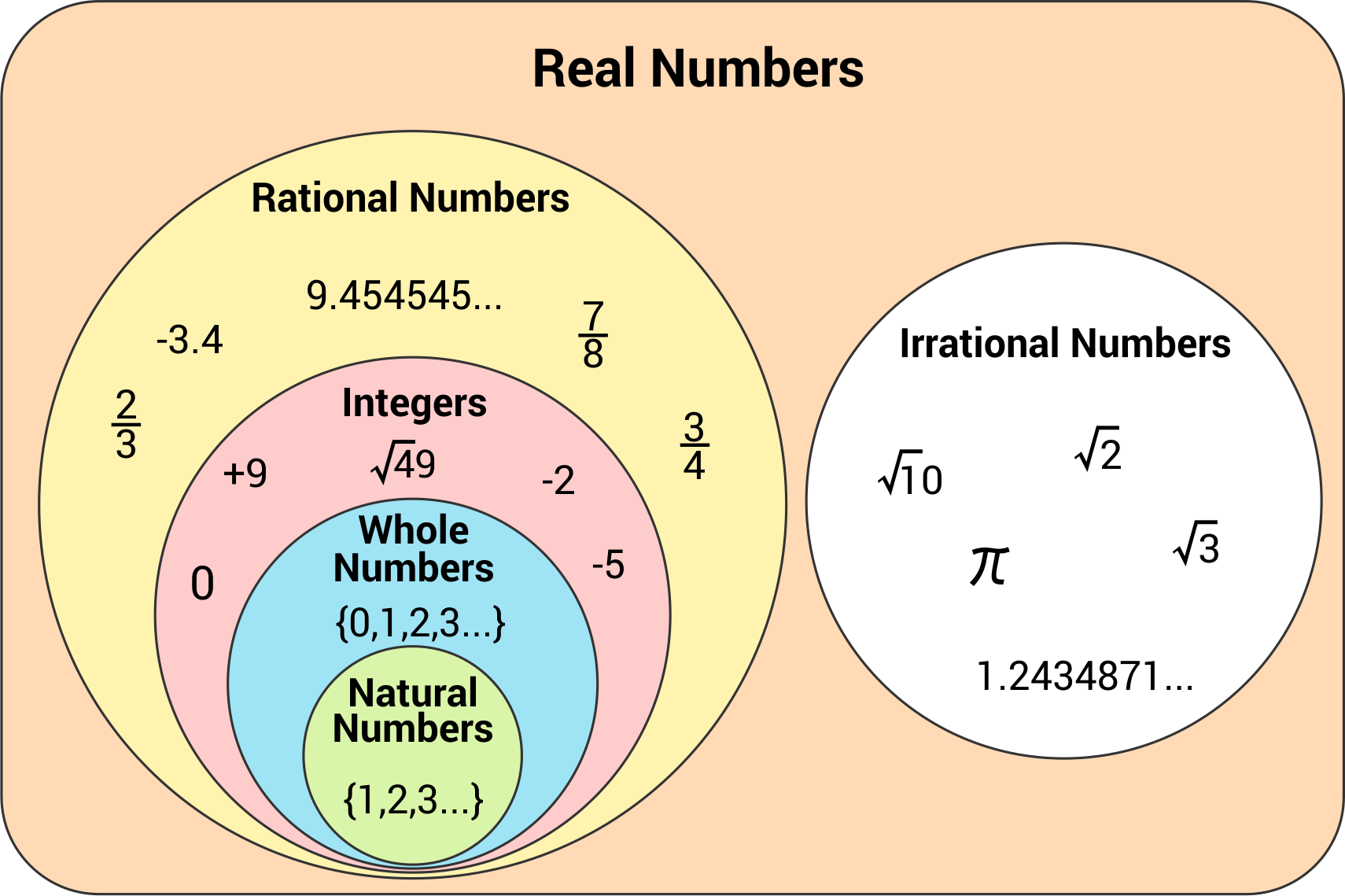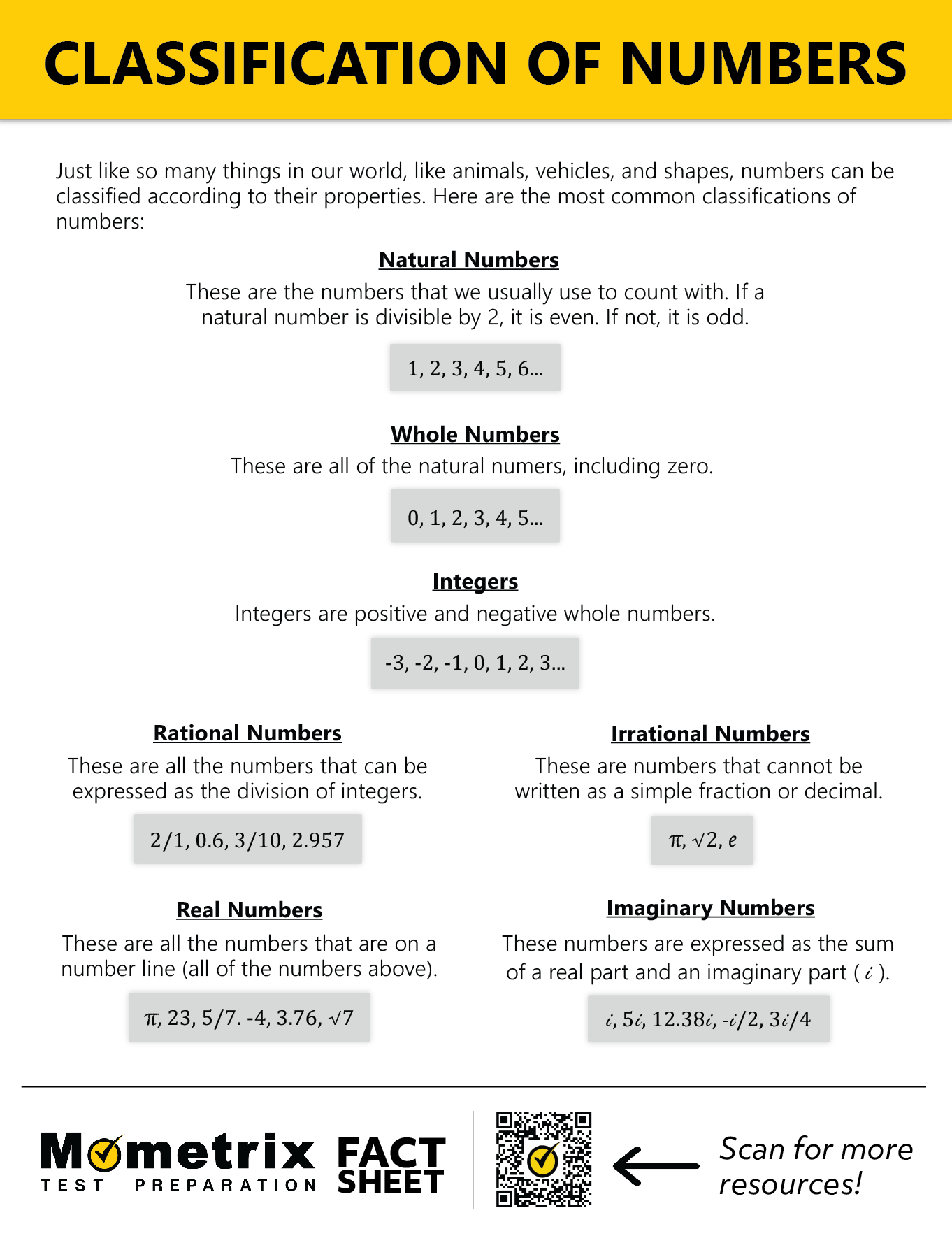# Classification of NumbersWhy do we classify numbers? Why do we give them names, like integers, irrational numbers, or negative numbers? For the same reason we classify anything, we want to make sure that everyone has an understanding of what specific numbers are called and what they mean. After all, there’s a difference between 25, and -32, and $$4^6$$.

## Types of Numbers

In this Mometrix video, we provide an overview of numbers and their classifications.

Numbers are our way of keeping order. We count the amount of money we have. We measure distance. We use percentages to indicate a sale. Numbers are an integral part of our everyday existence, whether they are whole numbers, rational numbers, or the first type of numbers we’re going to look at, real numbers.

## Real Numbers

A real number is any value of a continuous quantity that can represent distance on a number line. Essentially, it’s any number you can think of. Fifty (50) is a real number. One billion (1,000,000,000) is a very large real number. Real numbers encompass three classifications of numbers, which we’ll talk about in a little bit. Whole numbers, rational numbers, and irrational numbers are all real numbers.

## Imaginary Numbers

Imaginary numbers are not real numbers. They are complex numbers that are written as a real number multiplied by an imaginary unit ($$i$$). For instance, $$\sqrt{-1}$$ calculates as the imaginary number “$$i$$” and $$\sqrt{-25}=5i$$. Even though imaginary numbers aren’t “real numbers,” they do have value. Electricians use imaginary numbers when working with currents and voltage. Imaginary numbers are also used in complex calculus computations. So, just because these numbers are called “imaginary” doesn’t mean that they aren’t useful.

## Whole Numbers

Whole numbers are numbers we count with. 1, 2, 3, 4, and 5 are all whole numbers. So are -17 and 0. Whole numbers do not have fractions or decimals.

All whole numbers are called integers. Integers can be positive or negative whole numbers.

## Rational and Irrational Numbers

All integers and whole numbers are part of a bigger group called rational numbers. This group also includes fractions and decimals. That means $$\frac{3}{5}$$ and 7.25 are rational numbers. Rational numbers can also be positive or negative.

Rational numbers have opposites, which are called irrational numbers. These numbers can’t be written as a simple fraction. Pi ($$\pi$$) is the most famous irrational number. We have a close approximation of how to calculate pi, but it’s just a close approximation. Pi is renowned for going on forever. That’s why it’s an irrational number. You can’t easily write it as a fraction.

## Natural and Negative Numbers

Natural numbers are those that are positive integers, although there is some debate as to whether natural numbers start at 0 or 1. Negative numbers are, well, exactly that. They are the numbers below 0.

## Even and Odd Numbers

There are several other number classifications as well. Numbers are divided into even and odd numbers. If you can divide a number by 2, that number is even. So, 24, 36, and 74 are all even numbers because if you divide them by 2, you get 12, 18, and 37. Even numbers always end with 0, 2, 4, 6, or 8.

Odd numbers can’t be divided by 2 and leave a whole number. Any odd number divided by 2 will leave a fraction. So, 17 ÷ 2 = 8.5, and 23 ÷ 2 = 11.5. All odd numbers will end in 1, 3, 5, 7, or 9.

## Fractions

Numerators and denominators form fractions, which are comprised of two integers. The number on top is the numerator; the number on the bottom is the denominator. The numerator, the top number, shows how many parts we have. The denominator, the bottom number, shows how many parts make a whole.

Let’s say you have 6 apples and 3 of the apples get eaten. The number of apples you have left over would be displayed as $$\frac{3}{6}$$.

You would then divide 3, the top number, into 6, the bottom number, to determine the percentage of remaining apples. In this case, the number is 50%.

So that’s our look at numbers and their classifications. From whole numbers to irrational numbers, we need to know what to call numbers so we can know what they mean.

I hope this overview was helpful to you!

### What are the classifications of numbers?

#### A

The classifications of numbers are: real number, imaginary numbers, irrational number, integers, whole numbers, and natural numbers.

Real numbers are numbers that land somewhere on a number line.

Imaginary numbers are numbers that involve the number i, which represents $$\sqrt{-1}$$.

Rational numbers are any number that can be written as a fraction.

Irrational numbers are numbers that cannot be written as a fraction and include never-ending decimal numbers, like π.

Integers are numbers that do not have a fractional part, including positive and negative numbers and zero.

Whole numbers are positive integers and zero.

Natural numbers are positive integers and are sometimes called counting numbers.### How many classifications of real numbers are there?

#### A

There are 5 classifications of real numbers: rational, irrational, integer, whole, and natural/counting.

### How do you classify natural numbers?

#### A

Natural numbers, also called counting numbers, are positive integers. For example: 1, 2, 3, 4, . . .

### What is classified as a whole number?

#### A

A whole number is all natural/counting numbers and 0. For example: 0, 1, 2, 3, . . .

### What is the smallest whole number?

#### A

The smallest whole number is 0.

### What are counting numbers?

#### A

Counting numbers are positive integers. They are also called natural numbers. For example: 1, 2, 3, 4, . . .

### How do I classify real numbers?

#### A

Classify a real number as a rational number if it is able to be written as a fraction. For example: 7 $$(\frac{7}{1})$$, 6.25 $$(6\frac{1}{4}\text{ or}\frac{25}{4})$$, and $$\frac{11}{21}$$.

Classify a real number as an irrational number if it cannot be written as a fraction. For example: π, e, and $$\sqrt{2}$$. Classify a real number as an integer if it has no fractional part. For example: -2, 7, 0, and 24.

Classify a real number as a whole number if it is 0 or a positive integer. For example: 0, 1, 3, and 11.

Classify a real number as a natural number (or counting number) if it is a positive integer. For example: 1, 9, and 42.

## Classifying Numbers PDF## Practice Questions

Question #1:

The number 4 is not included in which group of numbers?

Integers

Whole numbers

Irrational numbers

Natural numbers

The correct answer is irrational numbers. Irrational numbers are numbers that cannot be written as a simple fraction or decimal, like π. Natural numbers are counting numbers: 1, 2, 3, 4 . . . Whole numbers are natural numbers and zero, and integers are positive and negative whole numbers.

Question #2:

What classification is the number i?

Real number

Irrational number

Imaginary number

Integer

The letter i is used to represent the imaginary number $$\sqrt{-1}$$.

Question #3:

What is the difference between a whole number and an integer?

Whole numbers can only be zero or positive natural numbers, but integers can also be negative natural numbers

Whole numbers are numbers that have no fractional part, and integers are fractions or decimal numbers

Whole numbers do not include zero, but integers do contain zero

Whole numbers have no imaginary part, but integers can have an imaginary part

Whole numbers are the set of all natural numbers including zero, and integers are positive and negative whole numbers. Neither whole numbers nor integers have a fractional or imaginary part.

Question #4:

Is 2.713 a rational number?

Yes

No

Sometimes

Cannot be determined

2.713 is a rational number. Rational numbers are all numbers that can be expressed as the division of integers. In other words, rational numbers are any number that can be represented by a fraction or a decimal.

Question #5:

Which of the following numbers is not odd?

21

73

49

16

16 is an even number, not an odd number. An odd number is any number that cannot be divided by 2.

461071454667

by Mometrix Test Preparation | This Page Last Updated: November 21, 2023

Get Actionable Study Tips
Join our newsletter to get the study tips, test-taking strategies, and key insights that high-performing students use.
Get Actionable Study Tips
Join our newsletter to get the study tips, test-taking strategies, and key insights that high-performing students use!